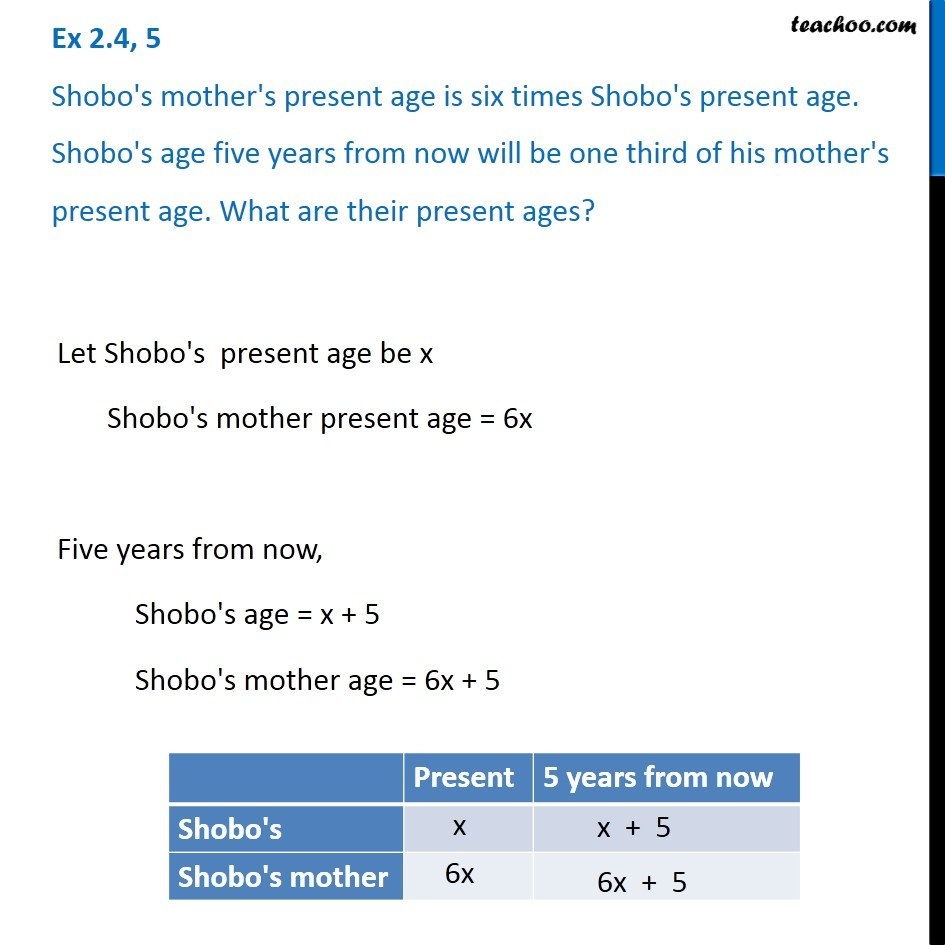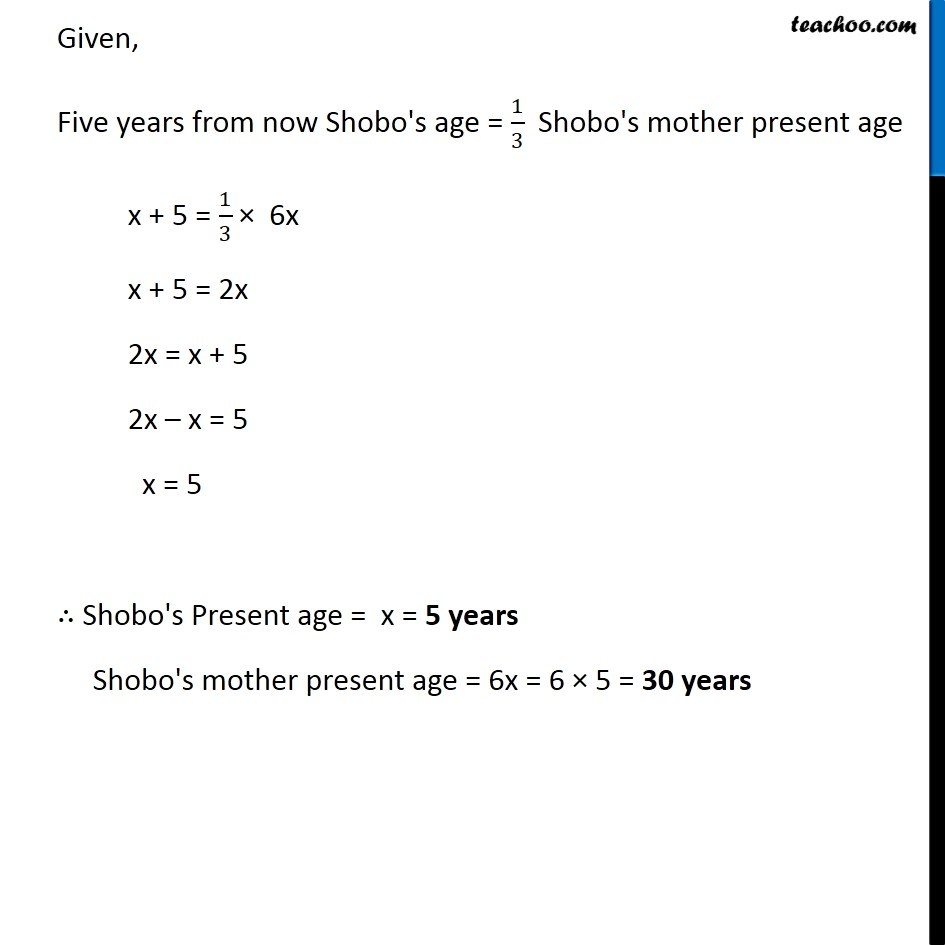Solving difficult equations

Chapter 2 Class 8 Linear Equations in One Variable
Serial order wiseLearn in your speed, with individual attention - Teachoo Maths 1-on-1 Class

### Transcript

Question 5 Shobo's mother's present age is six times Shobo's present age. Shobo's age five years from now will be one third of his mother's present age. What are their present ages?Let Shobo's present age be x Shobo's mother present age = 6x Five years from now, Shobo's age = x + 5 Shobo's mother age = 6x + 5 Given, Five years from now Shobo's age = 1/3 Shobo's mother present age x + 5 = 1/3 × 6x x + 5 = 2x 2x = x + 5 2x – x = 5 x = 5 ∴ Shobo's Present age = x = 5 years Shobo's mother present age = 6x = 6 × 5 = 30 years